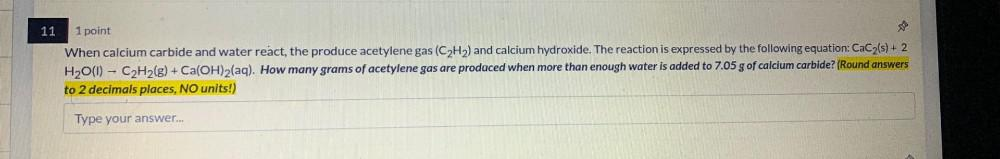Question:

# When calcium carbide and water react, the produce acetylene gas (C₂H₂) and calcium hydroxide. The reaction is expressed by the following equation: CaC₂(s) + 2 H₂O(l) --> C₂H₂(g) + Ca(OH)₂(aq). How manWhen calcium carbide and water react, the produce acetylene gas (C₂H₂) and calcium hydroxide. The reaction is expressed by the following equation: CaC₂(s) + 2 H₂O(l) --> C₂H₂(g) + Ca(OH)₂(aq). How many grams of acetylene gas are produced when more than enough water is added to 7.05 g of calcium carbide?